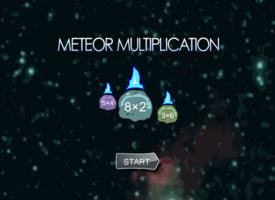Meteor Multiplication

 Meteor MultiplicationMeteor Multiplication assists students in learning multiplication. Large meteors with multiplication problems move toward a large space station in the center of the screen.

Tags:
math    meteor    multiplication    multiplication